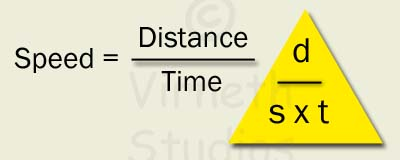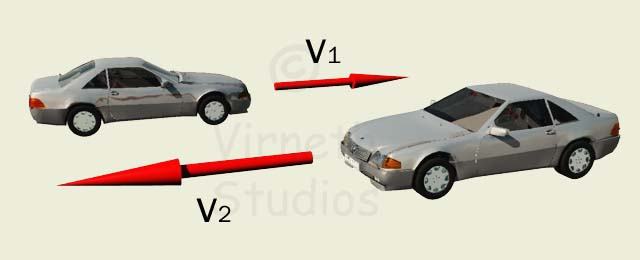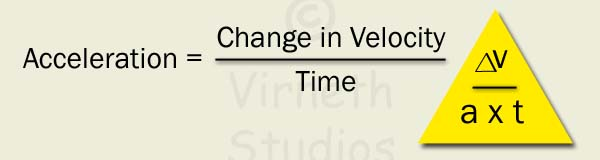# Speed, Velocity and Acceleration

## Speed

Speed is a measure of how fast an object is going or in other words how long it has taken to move a certain distance. It is given in m/s (metres per second), kph (kilometres per hour) or mph (miles per hour). A quantity with just a size (magnitude) Speed is a SCALAR because it only has a size (magnitude).## Velocity

Velocity is also a measure of how fast an object is travelling at, but unlike speed velocity is a VECTOR; the direction is important and makes a difference.

If two objects are both travelling at the same speed but in different directions then the velocities are different.Two cars at the same speed but in different direction means different velocities.

## Acceleration

Acceleration is a measure of how fast something is speeding up. Acceleration is stated in m/s2, or meters per second per second. This means the change of velocity (m/s) in one second.

Acceleration can be found using the expression : a= ∆v / t

Where

a = acceleration in m/s2
∆v = change in velocities (v2 - v1) in m/s
t = time in secondsAcceleration rates for some fast objects

 Object 0 - 100km/h (s) Acceleration (m/s2) Pistol Bullet at the end of the muzzle 0.00007 400,000 Dragster 0.7 40.0 Space Shuttle (max) 0.95 29.0 Bugatti Veyron Super Sport 2.4 11.57 Ariel Atom V8 2.5 11.1 Porsche 911 Turbo S 2.7 10.29 Ferrari Enzo 3.14 8.85 Space Shuttle (at take off) 5.0 5.56